# Boylestad: MCQ in Field Effect Transistor Devices

(Last Updated On: December 2, 2019)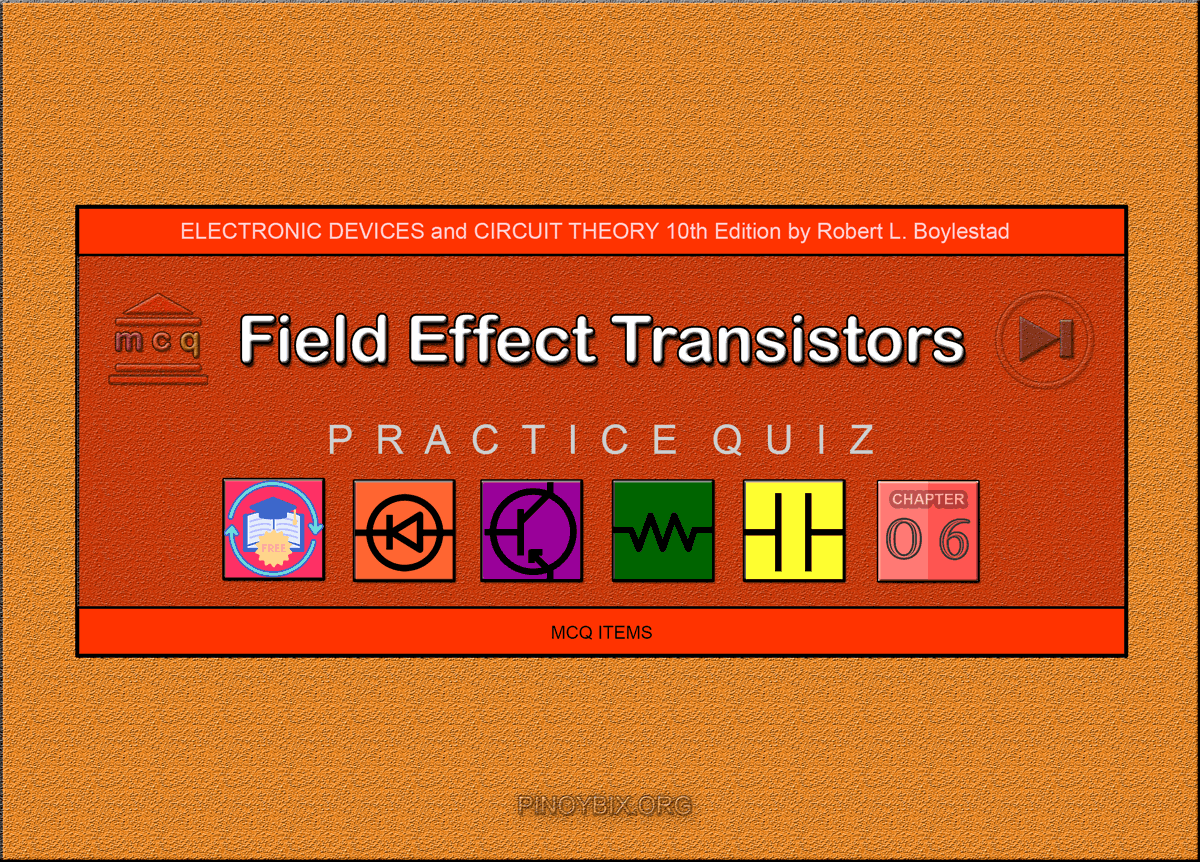This is the Multiple Choice Questions in Field Effect Transistor Devices from the book Electronic Devices and Circuit Theory 10th Edition by Robert L. Boylestad. If you are looking for a reviewer in Electronics Engineering this will definitely help. I can assure you that this will be a great help in reviewing the book in preparation for your Board Exam. Make sure to familiarize each and every questions to increase the chance of passing the ECE Board Exam.

#### Online Questions and Answers Topic Outline

• MCQ in FET Devices
• MCQ in Construction and Characteristics of JFETs
• MCQ in Transfer Characteristics
• MCQ in Specification Sheets (JFETs)
• MCQ in Instrumentation
• MCQ in Important Relationships
• MCQ in Depletion-Type MOSFET
• MCQ in Enhancement-Type MOSFET
• MCQ in MOSFET Handling
• MCQ in VMOS
• MCQ in CMOS

#### Practice Exam Test Questions

Choose the letter of the best answer in each questions.

1. Which of the following controls the level of ID?

A) VGS

B) VDS

C) IG

D) VDG

Solution:

2. Which of the following is (are) not an FET?

A) n-channel

B) p-channel

C) p-n channel

D) n-channel and p-channel

Solution:

3. What is the range of an FET’s input impedance?

A) 10 Ω to 1 kΩ

B) 1 kΩ to 10 kΩ

C) 50 kΩ to 100 kΩ

D) 1 MΩ to several hundred MΩ

Solution:

4. Which of the following transistor(s) has (have) depletion and enhancement types?

A) BJT

B) JFET

C) MOSFET

D) None of the above

Solution:

5. A BJT is a _______-controlled device. The JFET is a _______ – controlled device.

A) voltage, voltage

B) voltage, current

C) current, voltage

D) current, current

Solution:

6. The BJT is a _______ device. The FET is a _______ device.

A) bipolar, bipolar

B) bipolar, unipolar

C) unipolar, bipolar

D) unipolar, unipolar

Solution:

7. Which of the following is (are) the terminal(s) of a field-effect transistor (FET).

A) Drain

B) Gate

C) Source

D) All of the above

Solution:

8. What is the level of IG in an FET?

A) Zero amperes

B) Equal to ID

C) Depends on VDS

D) Undefined

Solution:

9. At which of the following is the level of VDS equal to the pinch-off voltage?

A) When ID becomes equal to IDSS

B) When VGS is zero volts

C) IG is zero

D) All of the above

Solution:

10. At which of the following condition(s) is the depletion region uniform?

A) No bias

B) VDS > 0 V

C) VDS = VP

D) None of the above

Solution:

11. Refer to the following characteristic curve. Calculate the resistance of the FET at VGS = –0.25 V if ro = 10 kΩ.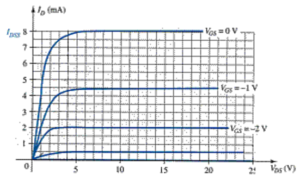A) 1.1378 kΩ

B) 113.78 Ω

C) 11.378 Ω

D) 11.378 kΩ

Solution:

12. What is the level of drain current ID for gate-to-source voltages VGS less than (more negative than) the pinch-off level?

A) zero amperes

B) IDSS

C) Negative value

D) Undefined

Solution:

13. The three terminals of the JFET are the ______, ______, and ______.

A) gate, collector, emitter

B) base, collector, emitter

C) gate, drain, source

D) gate, drain, emitter

Solution:

14. The level of VGS that results in ID = 0 mA is defined by VGS = _______.

A) VGS(off)

B) VP

C) VDS

D) None of the above

Solution:

15. The region to the left of the pinch-off locus is referred to as the _______ region.

A) saturation

B) cutoff

C) ohmic

D) All of the above

Solution:

16. Which of the following represent(s) the cutoff region for an FET?

A) ID = 0 mA

B) VGS = VP

C) IG = 0

D) All of the above

Solution:

17. Referring to this transfer curve. Calculate (using Shockley’s equation) VGS at ID = 4 mA.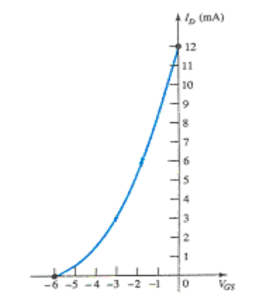A) 2.54 V

B) –2.54 V

C) –12 V

D) Undefined

Solution:

18. Referring to this transfer curve, determine ID at VGS = 2 V.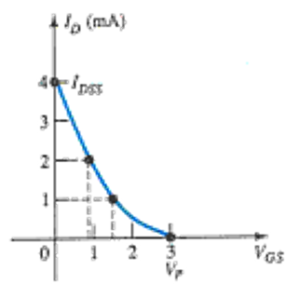A) 0.444 mA

B) 1.333 mA

C) 0.111 mA

D) 4.444 mA

Solution:

19. What is the ratio of ID/IDSS for VGS = 0.5 VP?

A) 0.25

B) 0.5

C) 1

D) 0

Solution:

20. The drain current will always be one-fourth of IDSS as long as the gate-to-source voltage is _______ the pinch-off value.

A) one-fourth

B) one-half

C) three-fourths

D) None of the above

Solution:

21. Which of the following ratings appear(s) in the specification sheet for an FET?

A) Voltages between specific terminals

B) Current levels

C) Power dissipation

D) All of the above

Solution:

22. Refer to this portion of a specification sheet. Determine the values of reverse-gate-source voltage and gate current if the FET was forced to accept it.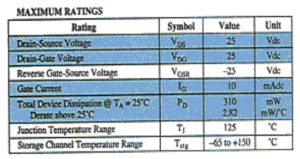D) None of the above

Solution:

23. Hand-held instruments are available to measure _______ for the BJT.

A) βdc

B) IDSS

C) VP

D) All of the above

Solution:

24. How many terminals can a MOSFET have?

A) 2

B) 3

C) 4

D) 3 or 4

Solution:

25. Which of the following applies to MOSFETs?

A) No direct electrical connection between the gate terminal and the channel

B) Desirable high input impedance

C) Uses metal for the gate, drain, and source connections

D) All of the above

Solution:

26. Referring to the following transfer curve, determine the level of VGS when the drain current is 20 mA.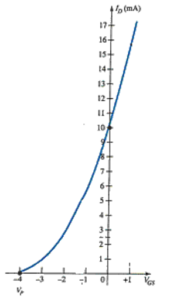A) 1.66 V

B) –1.66 V

C) 0.66 V

D) –0.66 V

Solution:

27. Refer to the following curves. Calculate ID at VGS = 1 V.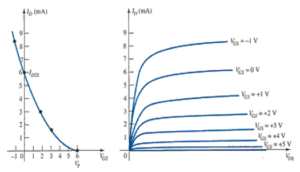A) 8.167 mA

B) 4.167 mA

C) 6.167 mA

D) 0.616 mA

Solution:

28. It is the insulating layer of ________ in the MOSFET construction that accounts for the very desirable high input impedance of the device.

A) SiO

B) GaAs

C) SiO2

D) HCl

Solution:

29. Refer to the following figure. Calculate VGS at ID = 8 mA for k = 0.278 × 10–2 A/V2.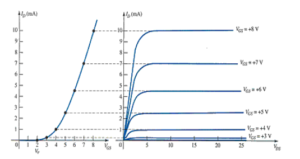A) 3.70 V

B) 5.36 V

C) 7.36 V

D) 2.36 V

Solution:

30. The transfer curve is not defined by Shockley’s equation for the ________.

A) JFET

B) depletion-type MOSFET

C) enhancement-type MOSFET

D) BJT

Solution:

31. Which of the following applies to a safe MOSFET handling?

A) Always pick up the transistor by the casing.

B) Power should always be off when network changes are made.

C) Always touch ground before handling the device.

D) All of the above

Solution:

32. What is the purpose of adding two Zener diodes to the MOSFET in this figure?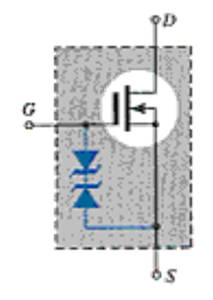A) To reduce the input impedance

B) To protect the MOSFET for both polarities

C) To increase the input impedance

D) None of the above

Solution:

33. Which of the following is (are) the advantage(s) of VMOS over MOSFETs?

A) Reduced channel resistance

B) Higher current and power ratings

C) Faster switching time

D) All of the above

Solution:

34. Which of the following FETs has the lowest input impedance?

A) JFET

B) MOSFET depletion-type

C) MOSFET enhancement-type

D) None of the above

Solution:

35. Which of the following input impedances is not valid for a JFET?

A) 1010 Ω

B) 109 Ω

C) 108 Ω

D) 1011 Ω

Solution:

#### Fill-in-the-blanks Questions

1. A junction field-effect transistor (JFET) is a ________ device.

A) current-controlled

B) voltage-controlled

C) voltage-current controlled

D) None of the above

Solution:

2. The FET is a ________ device depending solely on either electron (n-channel) or hole (p-channel) conduction.

A) unipolar

B) bipolar

C) tripolar

D) None of the above

Solution:

3. One of the most important characteristics of the FET is its _________ impedance.

A) low input

B) medium input

C) high input

D) None of the above

Solution:

4. The _________ transistor has become one of the most important devices used in the design and construction of integrated circuits for digital computers.

A) MOSFET

B) BJT

C) JFET

D) None of the above

Solution:

5. In the n-channel transistor, the drain and source are connected to the _______ channel while the gate is connected to the two layers of _______ material.

A) p-type, n-type

B) p-type, p-type

C) n-type, p-type

D) n-type, n-type

Solution:

6. In an FET transistor, the depletion region is ________ near the top of both p-type materials.

A) wider

B) narrower

C) the same as the rest of the depletion region

D) None of the above

Solution:

7. The pinch-off voltage continues to drop in a ________ manner as VGS becomes more and more negative.

A) linear

B) parabolic

C) cubic

D) None of the above

Solution:

8. The region to the right of the pinch-off locus is commonly referred to as the ________ region.

A) constant-current

B) saturation

C) linear amplification

D) All of the above

Solution:

9. As VGS becomes ________ negative, the slope of each curve in the characteristics becomes ________ horizontal corresponding with an increasing resistance level.

A) less, more

B) more, less

C) more, more

D) None of the above

Solution:

10. The transfer curve can be obtained by ________.

A) using Shockley’s equation

B) using both Shockley’s equation and by output characteristics

C) characteristics

D) None of the above

Solution:

11. The active region of an FET is bounded by ________.

A) ohmic region

B) cutoff region

C) power line

D) All of the above

Solution:

12. A(n) ________ can be used to check the condition of an FET.

A) digital display meter (DDM)

B) ohmmeter (VOM)

C) curve tracer

D) All of the above

Solution:

13. In a curve tracer, the ________ reveals the distance between the VGS curves for the n-channel device.

A) vertical sens.

B) horizontal sens.

C) Per step

D) gm

Solution:

14. In an FET circuit, ________ is normally the parameter to be determined first.

A) VGS

B) VDS

C) VDG

D) ID

Solution:

15. The primary difference between the construction of a MOSFET and an FET is the ________.

A) construction of the gate connection

B) low input impedance

C) threshold voltage

D) None of the above

Solution:

16. The primary difference between the construction of depletion-type and enhancement-type MOSFETs is ________.

A) the size of the transistor

B) the absence of the channel

C) the reverse bias junction

D) All of the above

Solution:

17. The level of ________ that results in the significant increase in drain current in enhancement-type MOSFETs is called threshold voltage VT’.

A) VDD

B) VDS

C) VGS

D) VDG

Solution:

18. In an n-channel enhancement-type MOSFET with a fixed value of VT’, the _______ the level of VGS’, the _____ the saturation level for VDS’.

A) higher, more

B) higher, less

C) lower, lower

D) None of the above

Solution:

19. The enhancement-type MOSFET is in the cutoff region if ________.

A) applied VGS is larger than VGS(Th)

B) applied VGS is less than or equal to VGS(Th)

C) VGS has a positive level

D) None of the above

Solution:

20. The specification sheet provides _________ to calculate the value of k for enhancement-type MOSFETs.

A) VGS(on)

B) ID(on)

C) VGS(Th)

D) All of the above

Solution:

21. ________ has high input impedance, fast switching speeds, and lower operating power levels.

A) CMOS

B) FET

C) BJT

D) None of the above

Solution:

22. The FET resistance in the ohmic region is ________ at VP and ________ at the origin.

A) smallest, largest

B) largest, smallest

C) larger, smaller

D) smaller, larger

Solution:

23. The silicon dioxide (SiO2) layer used in a MOSFET is _________.

A) an insulator

B) a conductor

C) a semiconductor

D) None of the above

Solution:

24. In an n-channel depletion-type MOSFET the region of positive gate voltages on the drain or transfer characteristics is referred to as the _________ region with the region between cutoff and the saturation level of ID referred to as the ________ region.

A) depletion, enhancement

B) enhancement, enhancement

C) enhancement, depletion

D) None of the above

Solution:

25. VMOS FETs have a ________ temperature coefficient that will combat the possibility of thermal runaway.

A) positive

B) negative

C) zero

D) None of the above

Solution:

### Complete List of Chapter Quiz in Electronic Devices and Circuit Theory

Help Me Makes a Difference!

 P inoyBIX educates thousands of reviewers/students a day in preparation for their board examinations. Also provides professionals with materials for their lectures and practice exams. Help me go forward with the same spirit. “Will you make a small \$5 gift today?” Option 1 : \$1 USD Option 2 : \$3 USD Option 3 : \$5 USD Option 4 : \$10 USD Option 5 : \$25 USD Option 6 : \$50 USD Option 7 : \$100 USD Option 8 : Other Amount© 2014 PinoyBIX Engineering. © 2019 All Rights Reserved | How to Donate? |#### GEAS Solution

Dynamics problem Economics problem Physics problem Statics problem Strength problem Thermodynamics problem

#### Questions and Answers in GEAS

Engineering Economics Engineering Laws and Ethics Engineering Management Engineering Materials Engineering Mechanics General Chemistry Physics Strength of Materials Thermodynamics
Consider Simple Act of Caring!: LIKE MY FB PAGE

Our app is now available on Google Play, Pinoybix Elex